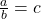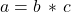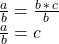## Which of the following is the correct equation we get when we rearrange a = b *c so that c is by itself on the right side of the

Question

Which of the following is the correct equation we get
when we rearrange a = b *c so that c is by itself on the
right side of the equals sign?
Oa=c
O a + b = c
O a/buc
O a*b = c

in progress 0
6 months 2021-08-04T13:00:54+00:00 1 Answers 8 views 0which agrees with the third answer in your list of answer options